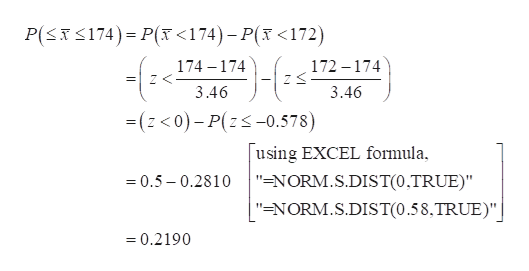# The average price of a college math textbook is \$174 and the standard deviation is \$23. Suppose that 44 textbooks are randomly chosen. Round all answers to 4 decimal places where possible.What is the distribution of ¯xx¯? ¯xx¯ ~ N(,)For the group of 44, find the probability that the average price is between \$172 and \$174. Find the first quartile for the average textbook price for this sample size. \$ (round to the nearest cent)

Question
1 views

The average price of a college math textbook is \$174 and the standard deviation is \$23. Suppose that 44 textbooks are randomly chosen. Round all answers to 4 decimal places where possible.

1. What is the distribution of ¯xx¯? ¯xx¯ ~ N(,)
2. For the group of 44, find the probability that the average price is between \$172 and \$174.
3. Find the first quartile for the average textbook price for this sample size. \$ (round to the nearest cent)
check_circle

Step 1

1.

In this context, it is given that the average price of a college math textbook is \$174 corresponding to its standard deviation of \$23.

Denote X as the speed for a randomly selected car.

For a random sample of 44, X follows normal with mean of the sampling distribution value of \$174 and standard deviation of \$3.46. That is X~N(174, 23/SQRT(44)).

Step 2

2.

The probability that the average price betw...help_outlineImage TranscriptioncloseP(xS174) P( <174)-P( <172) 174 174 172-174 2 3.46 3.46 -(z<0)-P(zs-0.578) using EXCEL formula "=NORM.S.DIST(0,TRUE)" 0.5-0.2810 "=NORM.S.DIST(0.58,TRUE)" 0.2190 fullscreen

### Want to see the full answer?

See Solution

#### Want to see this answer and more?

Solutions are written by subject experts who are available 24/7. Questions are typically answered within 1 hour.*

See Solution
*Response times may vary by subject and question.
Tagged in

### Hypothesis Testing